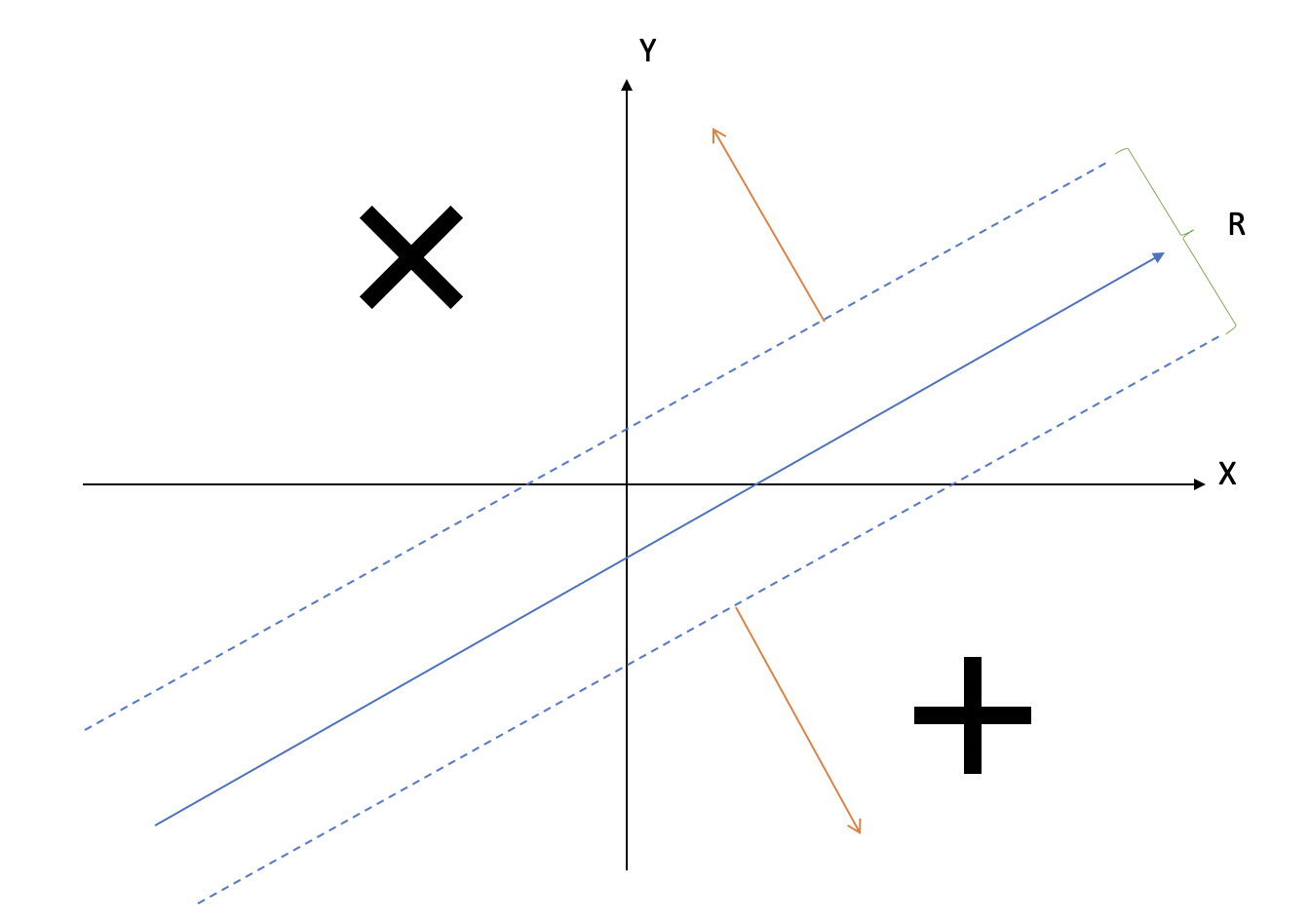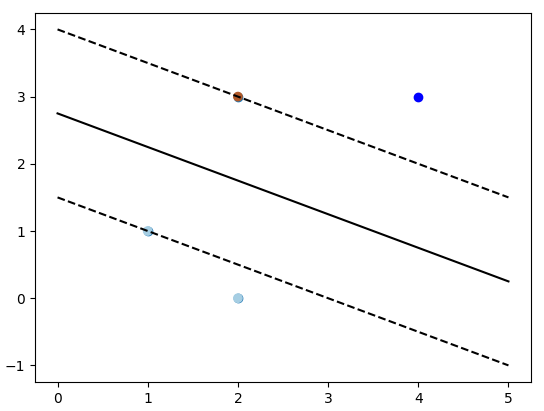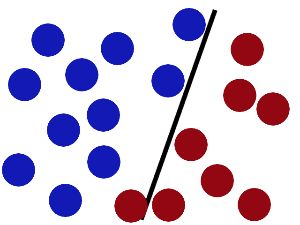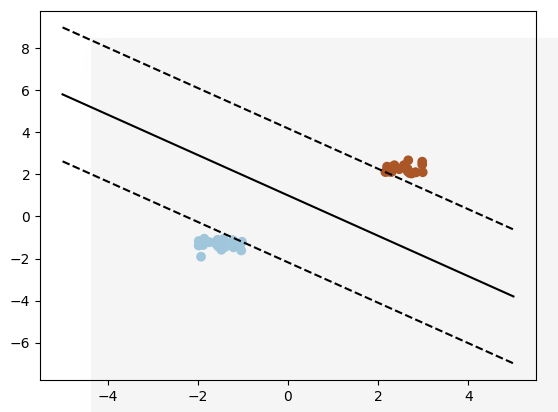• Address ...发现向量 (x,y)(x,y)(x,y) 和向量 (a,b)(a,b)(a,b) 点积，就等于过点 (x,y)(x,y)(x,y) 、斜率为 −ab-\frac ab−ba​ 直线 yyy 轴上截距 bbb 倍。 当 b&amp;gt;0b&amp;g...
Address
洛谷P3309
BZOJ3533
LOJ#2197
Solution
先假设询问对象是所有的向量，并且已经全部加入集合。
发现向量 $(x,y)$ 和向量 $(a,b)$ 的点积，就等于过点 $(x,y)$ 的、斜率为 $-\frac ab$ 的直线在 $y$ 轴上截距的 $b$ 倍。
当 $b>0$ 时，要最大化截距，所以要在集合内所有点的上凸壳上二分找到答案。
当 $b<0$ 时，要最小化截距，所以要在集合内所有点的下凸壳上二分。
当 $b=0$ 时，我们只需要最大化 $ax$ ，所以在上凸壳和下凸壳都行。
如果加入「第 $L$ 个到第 $R$ 个加入的向量」这一限制，又怎么做呢？
线段树！！！！！
线段树每个节点维护对应区间内的点构成的上下凸壳。
但还有一个困难：每次加入的点的 $x$ 坐标没有单调性，所以不能直接增量维护凸壳，而如果每一次更新都将某个节点 $[l,r]$ ，合并 $[l,mid]$ 和 $[mid+1,r]$ 的上下凸壳到 $[l,r]$ ，那么插入的复杂度将是 $O(n)$ 的，没有任何改进。
但继续思考，我们只需要在末尾加向量而不是修改一个向量，可以怎样优化？
发现：对于每个线段树上的节点对应的区间 $[l,r]$ ，如果已经加入的向量数 $T<r$ ，那么这个点在加入其他向量之前一定不会被使用到。
所以，我们的策略是：线段树上的节点 $p$ ，如果 $p$ 对应的区间为 $[l,r]$ ，当且仅当 $T=r$ 时才从 $p$ 的两个子节点合并。
这样，每个节点都只进行了一次合并。
查询时只需要找到区间 $[L,R]$ 在线段树上拆成的不超过 $O(\log n)$ 个区间后在这些区间的 上 / 下 凸壳上二分查找最大值即可。
时间复杂度 $O(n\log^2n)$ 。
Code
#include <cmath>
#include <cstdio>
#include <vector>
#include <cstring>
#include <iostream>
#include <algorithm>
#define For(i, a, b) for (i = a; i <= b; i++)
#define p2 p << 1
#define p3 p << 1 | 1

{
int res = 0; bool bo = 0; char c;
while (((c = getchar()) < '0' || c > '9') && c != '-');
if (c == '-') bo = 1; else res = c - 48;
while ((c = getchar()) >= '0' && c <= '9')
res = (res << 3) + (res << 1) + (c - 48);
return bo ? ~res + 1 : res;
}

inline char get()
{
char c;
while ((c = getchar()) != 'A' && c != 'Q');
return c;
}

template <class T>
inline T Max(const T &a, const T &b) {return a > b ? a : b;}

typedef long long ll;

const int N = 4e5 + 5, M = N << 2;

int n, T;

struct point
{
int x, y;

friend inline point operator - (point a, point b)
{
return (point) {b.x - a.x, b.y - a.y};
}

friend inline ll operator * (point a, point b)
{
return 1ll * a.x * b.y - 1ll * a.y * b.x;
}
};

std::vector<point> up[M], dn[M];
bool isfull[M];
char s[N];

{
int top = up[p].size() - 1;
while (top > 0 && (up[p][top - 1] - up[p][top]) * (up[p][top - 1] - x) >= 0)
top--, up[p].pop_back();
up[p].push_back(x);
}

{
int top = dn[p].size() - 1;
while (top > 0 && (dn[p][top - 1] - dn[p][top]) * (dn[p][top - 1] - x) <= 0)
top--, dn[p].pop_back();
dn[p].push_back(x);
}

void merge_up(int p)
{
int i, n1 = up[p2].size(), n2 = up[p3].size(), q1 = 0, q2 = 0;
For (i, 1, n1 + n2)
if (q2 == n2 || (q1 < n1 &&
(up[p2][q1].x < up[p3][q2].x || (up[p2][q1].x == up[p3][q2].x
&& up[p2][q1].y < up[p3][q2].y))))
}

void merge_dn(int p)
{
int i, n1 = dn[p2].size(), n2 = dn[p3].size(), q1 = 0, q2 = 0;
For (i, 1, n1 + n2)
if (q2 == n2 || (q1 < n1 &&
(dn[p2][q1].x < dn[p3][q2].x || (dn[p2][q1].x == dn[p3][q2].x
&& dn[p2][q1].y < dn[p3][q2].y))))
}

ll findmax_up(int p, int a, int b)
{
int l = 0, r = up[p].size() - 2;
while (l <= r)
{
int mid = l + r >> 1;
if (1ll * up[p][mid].x * a + 1ll * up[p][mid].y * b
>= 1ll * up[p][mid + 1].x * a + 1ll * up[p][mid + 1].y * b)
r = mid - 1;
else l = mid + 1;
}
return 1ll * up[p][l].x * a + 1ll * up[p][l].y * b;
}

ll findmax_dn(int p, int a, int b)
{
int l = 0, r = dn[p].size() - 2;
while (l <= r)
{
int mid = l + r >> 1;
if (1ll * dn[p][mid].x * a + 1ll * dn[p][mid].y * b
>= 1ll * dn[p][mid + 1].x * a + 1ll * dn[p][mid + 1].y * b)
r = mid - 1;
else l = mid + 1;
}
return 1ll * dn[p][l].x * a + 1ll * dn[p][l].y * b;
}

void addpoint(int l, int r, int pos, point x, int p)
{
if (l == r)
{
up[p].push_back(x); dn[p].push_back(x);
return (void) (isfull[p] = 1);
}
int mid = l + r >> 1;
if (pos <= mid) addpoint(l, mid, pos, x, p2);
else addpoint(mid + 1, r, pos, x, p3);
if (isfull[p2] && isfull[p3])
merge_up(p), merge_dn(p), isfull[p] = 1;
}

ll querymax(int l, int r, int s, int e, int a, int b, int p)
{
if (l == s && r == e)
return b >= 0 ? findmax_up(p, a, b) : findmax_dn(p, a, b);
int mid = l + r >> 1;
if (e <= mid) return querymax(l, mid, s, e, a, b, p2);
else if (s >= mid + 1) return querymax(mid + 1, r, s, e, a, b, p3);
else return Max(querymax(l, mid, s, mid, a, b, p2),
querymax(mid + 1, r, mid + 1, e, a, b, p3));
}

int main()
{
int i, l, r, x, y; ll lst = 0; char op;
n = read(); scanf("%s", s + 1);
For (i, 1, n)
{
if (s != 'E') x = x ^ (lst & 0x7fffffff),
y = y ^ (lst & 0x7fffffff);
if (op == 'A') T++, addpoint(1, n, T, (point) {x, y}, 1);
else
{
if (s != 'E')
{
l = l ^ (lst & 0x7fffffff);
r = r ^ (lst & 0x7fffffff);
}
printf("%lld\n", lst = querymax(1, n, l, r, x, y, 1));
}
}
return 0;
}



展开全文凸包 线段树
• ans/y表示过点(z,w)的斜率为-x/y的直线在y轴上的截距 当y>0时，截距越大，ans越大，在上凸壳上找答案 当y 答案可以三，也可以直接set维护斜率找斜率最接近的那个点 问题来了，如何维护区间[L,R]的凸包 ...
考虑一个查询操作
xz+yw=ans
w+x/y*z=ans/y
w=-x/y*z+ans/y
ans/y表示过点(z,w)的斜率为-x/y的直线在y轴上的截距
当y>0时，截距越大，ans越大，在上凸壳上找答案
当y<0时，截距越小，ans越大，在下凸壳上找答案
答案可以三分，也可以直接set维护斜率找斜率最接近的那个点
问题来了，如何维护区间[L,R]的凸包
线段树的每个节点建凸包是O(size log n)的
所以把所有的节点都建出凸包是O(∑size log n)的
因为O(∑size)=O(n log n)一层有n个点，有log n层
所以总复杂度是O(n log^2 n)的，空间复杂度是O(n log n)的
但是，题目要求在线插入，也就是说不能预处理出整棵线段树
如果暴力插入，每次要修改O(log n)个节点，每个节点复杂度为O(size)的，每次修改的复杂度是O(nlogn)。
如果每个节点用平衡树来维护的话是O(log size)的，复杂度就是O(log^2 n)的
我们发现，如果当前插入的节点为i，那么有一些节点是现在不会询问到的，那么就并不需要更新
每个节点打一个标记表示是否维护过，对于新插入节点的祖先，如果包含不会询问的节点，就不新建
因为每个节点只会被新建一次，所以复杂度是O(nlog^2n)的
或者，有更优的方法，我们何必建出那些不会被询问的节点呢？
所以，每次询问的时候再去新建就可以，复杂度也是O(nlog^2n)的

我还真是日了狗，第二次了，本机AC，bz上RE，求解释呀！！！

#include<cstdio>
#include<cstring>
#include<cstdlib>
#include<cmath>
#include<algorithm>
#include<iostream>
#include<vector>
#define maxn 500010
#define inf 1000000000000000000ll

using namespace std;

struct yts2
{
long long x,y;
}p[maxn];

long long operator*(yts2 a,yts2 b)
{
return a.x*b.y-a.y*b.x;
}

long long operator^(yts2 a,yts2 b)
{
return a.x*b.x+a.y*b.y;
}

yts2 operator-(yts2 x,yts2 y)
{
yts2 ans;
ans.x=x.x-y.x;ans.y=x.y-y.y;
return ans;
}

struct yts
{
int l,r;
bool flag;
int sizeup,sizedw;
vector<int> up,dw;
}t[4*maxn];

struct yts1
{
int op;
int x,y;
int l,r;
}q[maxn];

int decode(int x,long long ans)
{
return x^(ans&0x7fffffff);
}

bool cmp(int i,int j)
{
return p[i].x<p[j].x || (p[i].x==p[j].x && p[i].y<p[j].y);
}

int n,m,T,tot,tmp[maxn];
long long ans;
char s1,s;

void build(int i,int l,int r)
{
t[i].l=l;t[i].r=r;t[i].flag=0;
for (int j=l;j<=r+1;j++) t[i].up.push_back(0),t[i].dw.push_back(0);
if (l==r) return;
int mid=(l+r)/2;
build(i*2,l,mid);build(i*2+1,mid+1,r);
}

long long query(int i,long long x,long long y)
{
long long ans=-inf;
yts2 o;
o.x=x;o.y=y;
if (y>=0)
{
int l=1,r=t[i].sizeup;
while (r-l>=3)
{
int mid=l+(r-l)/3,midmid=r-(r-l)/3;
if ((o^p[t[i].up[mid]])>(o^p[t[i].up[midmid]])) r=midmid; else l=mid;
}
for (int j=l;j<=r;j++) ans=max(ans,o^p[t[i].up[j]]);
}
else
{
int l=1,r=t[i].sizedw;
while (r-l>=3)
{
int mid=l+(r-l)/3,midmid=r-(r-l)/3;
if ((o^p[t[i].dw[mid]])>(o^p[t[i].dw[midmid]])) r=midmid; else l=mid;
}
for (int j=l;j<=r;j++) ans=max(ans,o^p[t[i].dw[j]]);
}
return ans;
}

void build(int i)
{
int size=0;
for (int j=t[i].l;j<=t[i].r;j++) tmp[++size]=j;
sort(tmp+1,tmp+size+1,cmp);
t[i].sizeup=0;t[i].sizedw=0;
for (int j=1;j<=size;j++)
{
while (t[i].sizeup>1 && (p[tmp[j]]-p[t[i].up[t[i].sizeup]])*(p[t[i].up[t[i].sizeup]]-p[t[i].up[t[i].sizeup-1]])<=0) t[i].sizeup--;
t[i].up[++t[i].sizeup]=tmp[j];
while (t[i].sizedw>1 && (p[tmp[j]]-p[t[i].dw[t[i].sizedw]])*(p[t[i].dw[t[i].sizedw]]-p[t[i].dw[t[i].sizedw-1]])>=0) t[i].sizedw--;
t[i].dw[++t[i].sizedw]=tmp[j];
}
}

long long query(int i,int l,int r,long long x,long long y)
{
if (l<=t[i].l && t[i].r<=r)
{
if (!t[i].flag) t[i].flag=1,build(i);
return query(i,x,y);
}
int mid=(t[i].l+t[i].r)/2;
long long ans=-inf;
if (l<=mid) ans=max(ans,query(i*2,l,r,x,y));
if (mid<r) ans=max(ans,query(i*2+1,l,r,x,y));
return ans;
}

int main()
{
scanf("%d%s",&T,s1);
for (int i=1;i<=T;i++)
{
scanf("%s",s);
scanf("%d%d",&q[i].x,&q[i].y);
if (s=='A') q[i].op=1,n++;
else
{
q[i].op=2;
scanf("%d%d",&q[i].l,&q[i].r);
}
}
build(1,1,n);
for (int i=1;i<=T;i++)
{
if (s1!='E') q[i].x=decode(q[i].x,ans),q[i].y=decode(q[i].y,ans);
if (q[i].op==1) p[++tot].x=q[i].x,p[tot].y=q[i].y;
else
{
q[i].l=decode(q[i].l,ans);q[i].r=decode(q[i].r,ans);
ans=query(1,q[i].l,q[i].r,q[i].x,q[i].y);
printf("%lld\n",ans);
}
}
return 0;
}
展开全文• 假设样本能够分类，则必有一超...由表达式看出，支持向量（平面）平行于分类超平面，且是上下移动了1k个单位，k对于不同坐标具有不同参数，具体为W分量Wx或Wy倒数。 支持平面Y+，Y-： Y+：WT * X + b - 1 ...假设样本能够分类，则必有一超平面WTX + b将正负样本分开。
支持向量：
WT * X + b >= +1, y+ = +1;
WT * X + b <= -1 , y- = -1;
由表达式看出，支持向量（平面）平行于分类超平面，且是在上下移动了1k个单位，k对于不同的坐标轴具有不同的参数，具体为W分量Wx或Wy的倒数。
支持平面Y+，Y-：
Y+：WT * X + b - 1 = 0
Y-：WT * X + b + 1 = 0
因为两平面平行，则Y+上所有点X距离平面Y-都是相同的。
则利用点到直线(平面)公式，将点X带入平面Y-方程
即将WT * X = 1 - b 代入 WT * X + b + 1 = 0
r = | 1 - b + b - 1  | / ||W||
则间隔为：
R = 2 / ||W||


展开全文SVM
• 　如下图所示，x,y坐标轴上，我们绘制3个点A（1,1），B（2,0），C（2,3），其中A和B属于一类，C属于一类。 　我们希望找到一条直线，将两个类分开来，且保持实线和两条虚线距离最大，我们就能将两个类最大化...

学习了SVM分类器的简单原理，并调用sklearn库，对40个线性可分点进行训练，并绘制出图形画界面。
一、问题引入
如下图所示，在x,y坐标轴上，我们绘制3个点A（1,1），B（2,0），C（2,3），其中A和B属于一类，C属于一类。
我们希望找到一条直线，将两个类分开来，且保持实线和两条虚线的距离最大，我们就能将两个类最大化分割开来。当然，我们还有很多其他直线的可以将两个点分割开来，但是这样分割效果最好。
当一个新的点进行预测时，根据点在直线的位置，判断所属分类。例如D（4,3）点在实线上方，判定为和C是一类。
源码：# 导入基本库
from sklearn import svm
import pylab as pl
import numpy as np
# 初始化坐标点
x = np.zeros((3,2))
x = [2,0]
x = [1,1]
x = [2,3]
y = [0,0,1]
# 建立一个SVM分类器，并进行预测
clf = svm.SVC(kernel='linear')
clf.fit(x,y)

# 打印出所有的支持向量点
print(clf.support_vectors_)
# 根据方程w_0*x + w_1*y + w_3 = 0--> y = -w_0/w_1*x - w_3/w_1 = kx + b
# 根据w_0,w_1,w_3求得k和b
w = clf.coef_
k = -w/w
b = -clf.intercept_/w
# 调用函数，初始化x点坐标范围
xx = np.linspace(start=0, stop = 5)
# 计算直线方程
yy = k*xx + b

# 获取第一个支持向量点，计算直线方程
b = clf.support_vectors_
yy_down = k*xx + (b - k*b)

# 获取最后一个支持向量点，计算直线方程
b = clf.support_vectors_[-1]
yy_up = k*xx + (b - k*b)

# 绘制点和直线
pl.scatter(x[:,0],x[:,1])
pl.plot(xx,yy,'k-')
pl.plot(xx,yy_down,'k--')
pl.plot(xx,yy_up,'k--')
pl.scatter(x[:,0],x[:,1],c=y,cmap=pl.cm.Paired)
pl.scatter(4,3,c=y,cmap=pl.cm.Paired)
pl.show()

View Code二、SVM分类简单介绍
N. Vapnik 和 Alexey
Ya. Chervonenkis 在1963年提出，目前的版本(soft
margin)是由Corinna
Cortes 和
Vapnik在1993年提出，并在1995年发表，深度学习（2012）出现之前，SVM被认为机器学习中近十几年来最成功，表现最好的算法。
假设我们的测试数据为一个二维平面的点，如上图例子所示，我们定义一条直线方程为w_0*x
+ w_1*y + w_3 =
0，SVM算法的原理就是要根据训练集获取到参数w_0、w_1、w_3的值，即可利用该模型进行新的测试数据预测。对于参数的求导过程，可以参考下面链接：
https://www.zhihu.com/question/21094489
当我们无法找到一条直线，将不同类分割开来时，我们称之为线性不可分，否则为线性可分。例如下面所示为线性不可分的情况。对于线性不可分的情况，可以通过一定的映射关系，转换到其他平面，变成线性可分，例如二维左边转换为极坐标。上面讨论的是只有两种分类，如果遇到大于2中分类的情况，我们可以用迭代分类点方式进行，例如有A,B,C三类，我们可以先将A,B化为一类，整体和C进行分类，如果属于A,B整体类，再对A,B进行分类，以此类推。

三、简单的SVM代码实现
程序效果图：对40个线性可分点进行训练，并绘制出图形界面。# 函数功能：以[2,2]为中心，随机产生上下40个线性可分的点，画出支持向量和所有的点

# 导入基本库
import numpy as np
import pylab as pl
from sklearn import svm

# 每次程序运行时，产生的随机点都相同
np.random.seed(0)# 产生40行随机坐标，且线性可区分
x = np.r_[np.random.rand(20,2) - [2,2],np.random.rand(20,2) + [2,2]]
y = *20 + *20# 创建一个SVM分类器并进行预测
clf = svm.SVC(kernel='linear')
clf.fit(x,y)# 根据SVM分类器类参数，获取w_0,w_1，w3的值，并绘制出支持向量
# x_0*x + w_1 *y + w_3 = 0 --> y = -w0/w1*x - w_3/w_1
w = clf.coef_
a = -w/w
b = -clf.intercept_/w
xx = np.linspace(-5, 5)
yy = a*xx + b

# 斜距式方程：y = kx + b，A(b,b)为一个支持向量点
b = clf.support_vectors_
yy_down = a*xx + (b - a*b)

# 斜距式方程：y = kx + b，B(b,b)为一个支持向量点
b = clf.support_vectors_[-1]
yy_up = a*xx + (b - a*b)

#画出3条直线
pl.plot(xx,yy,'k-')
pl.plot(xx,yy_down,'k--')
pl.plot(xx,yy_up,'k--')

#画出支持向量点
pl.scatter(clf.support_vectors_[:,0], clf.support_vectors_[:,1],
s=80,facecolors = 'none')
pl.scatter(x[:,0],x[:,1],c=y,cmap=pl.cm.Paired)

# 绘制平面图
pl.axis('tight')
pl.show()

四、学习总结
在学习过程中，重新复习了直线方程的表达式：两点式、点斜式、斜距式、截距式，以及点到直线的距离等。同时了解numpy库对于数组的基本操作。

转载于:https://www.cnblogs.com/python-frog/p/8879079.html
展开全文• ## CDM(码分多路复用技术)总结

万次阅读 多人点赞 2016-09-29 21:29:43
多路复用是一种数学上的规律运用数据链路层。当想起CDM时，脑海中应该想起的是一个坐标系，简单的是二维平面直角坐标系，再复杂些是三维的空间直角坐标系。更高维的就难想象了。But, try it.为什么想到坐标系...网络 CDM
• ## 码分复用及其计算

万次阅读 多人点赞 2018-10-21 22:04:26
多路复用是一种数学上的规律运用数据链路层。 当想起CDM时，脑海中应该想起的是一个坐标系，简单的是二维平面直角坐标系，再复杂些是三维的空间直角坐标系。更高维的就难想象了。 为什么想到坐标系呢？  先...
• // 向量va初始值可以赋值为y轴的单位向量 va.x = 0; va.y = 1; // 寻找其它点 while( 1 ) { // 先给maxcos一个最小值，当有更大值出现时，对其进行修改 maxcos = -1; // cos(90) // 所有点中...c 凸包问题
• HDU - 4855 借这道题学了下极角排序和三求凸函数最大值 按所有左右切线，圆心的弧度值排序，从而分割出角度上的若干个区间 射线的角度这些区间里变动...2) 利用 atan2(y,x)可以比较方便地求出向量与 x正方向的
• Principal Component Analysis 基于方差查找方差最大方向，方差越大方向 数据更容易分开，数据分的越开 更易于对其分类。...向量(3,2)是在x轴上有个投影和在y轴上有个投影。 有了一组基之后，在x轴方向和...
• 首先将输入点(x,y)变为(-y,x)然后，只需找一个向量与(-y,x)的点积最大，即找一个向量在(-y,x)上的投影最长。此时所有的点都是在x上方的，容易发现答案一定是在凸包上的，再继续观察，如果有一个点在凸包而不在上...
• 学习支持向量过程中，我们知道其损失函数为合页损失函数。至于为什么叫这个名字，李航老师《统计学习方法》是这么说：由于函数形状像一个合页，故命合页损失函数。下图为合页损失函数图像（取自《统计...计算机视觉
• 矩阵是怎样变换向量的向量在几何能被解释成一系列与平行位移，一般来说，任意向量v都能写成“扩展”形式：另一种略有差别形式为：注意右边单位向量就是x，y，z，这里只是将概念数学化，向量每个坐标都...扩展 xp
• 其中X,Y,是平面几何坐标,Z是物理量(如平板上的压力) ----Gridding后 Map -> Vector Map -> New 1-Grid Vector 即可 ----这是最基本的平面坐标向量图,直接以物理量Z值为向量图依据. --b.如果数据是X,Y,Z,Z1 ----...
• 用于Arduino和LSM9DS1传感器的Mahony AHRS和Tilt Compensated Compass，用于Arduino和LSM9DS1传感器，使用Arduino Pro Mini上的I2C连接为Adafruit LSM9DS1支板编写和测试。 2021年3月更新：实施了一个新的Mahony...
• Jalpha查找表 14.3.3示例——将数据映射到颜色或透明度 14.4选择一...上的显示比率 第16章MATLAB提供的科学计算可视化工具 16.1剖面图 16.1.1slice函数 16.1.2切片等值线图 16.1.3切片流线图 ...
• fx = f/dx, fy = f/dy,分别称为x轴和y轴上的归一化焦距. 为更好的理解，举个实例： 现以NiKon D700相机为例进行求解其内参数矩阵： 就算大家身边没有这款相机也无所谓，可以网上百度一下，很方便的就知道其一些...matlab
• 本来应该是很简单，利用一个特征向量y轴的值）作为分类依据，将红色数据和蓝色数据分开。 然而，利用matlab建立BP神经网络，训练结果有时候非常好，测试正确率能达到98以上，有时候正确率只有20~30。 ...人工智能 机器学习 神经网络
• 其中X,Y,是平面几何坐标,Z是物理量(如平板上的压力) ----Gridding后 Map -> Vector Map -> New 1-Grid Vector 即可 ----这是最基本的平面坐标向量图,直接以物理量Z值为向量图依据. --b.如果数据是X,Y,Z,Z1 ----...
• ## surfer 9.11 汉化.part1

热门讨论 2011-03-23 08:46:07
其中X,Y,是平面几何坐标,Z是物理量(如平板上的压力) ----Gridding后 Map -> Vector Map -> New 1-Grid Vector 即可 ----这是最基本的平面坐标向量图,直接以物理量Z值为向量图依据. --b.如果数据是X,Y,Z,Z1 ----...